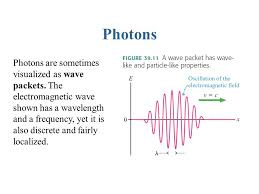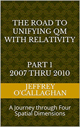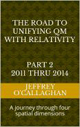# What is a photon?

Both Einstein’s Special Theory of Relativity and Quantum Mechanics tell us a photon is massless particle that moves through space. However, they tell us nothing about how a particle can be mass less, how they interact with other particles and how the photon a particle with no mass as defined in Quantum Electrodynamics or QED has the momentum to travel though space at the speed of light.

Einstein tells us that the mass of all entities including particles approaches infinity as it approaches the speed of light.  Therefore, according to him a photon has to be massless because if it wasn’t it could not attain that speed.  However, Quantum mechanics defined photons as the fundamental unit (quanta) of light while having the unique property of being both a particle and wave. They are often described as being mass less "bundles of pure energy traveling at the speed of light.However, as was just mentioned neither of these two well established and verified theories tell us what it is, how they move through space, how a particle mass less can move at the speed of light or how they interact with other particles that have mass.

Granted Quantum Electrodynamics (QED) describes how light and matter interact and is the first theory where full agreement between quantum mechanics and Special Relativity is achieved in terms of an exchange of photons and represents the quantum counterpart of classical electromagnetism giving a complete account of matter and light interaction.  However, it tells us nothing how a photon moves through space and the causality of the electromagnetic properties of its wave component which are a fundamental part of its definition.

Yet Einstein also was unable to define an electromagnetic wave and how it moves through space in terms of his Special and General Theories of Relativity even though he used electromagnetic energy to define their physical existence.

However, Einstein gave us a way to define what a photon is, derive its electromagnetic properties, how it moves thought space and interacts with matter in a manner which is not only consistent with his theories but also with our classical understanding of nature when, in his General Theory of Relativity he showed that matter can be converted into energy or energy into matter according to the equation E=mc2.

For example, Einstein defined the origin of the mass component of particles and all other objects, such as the sun in terms of curvature or distortion in the continuous field properties of space-time not in terms of its particle properties.  Specifically, he defined mass and its potential energy in terms of positive curvature in the spatial geometry of our universe which causes energy to become concentrated at the apex of that curvature.

However, in doing so he also defined the kinetic energy released when mass is converted to energy as reduction, an opposite or a negatively directed curvature in space-time. In other words when defined the potential energy of mass in terms of a positive curvature he indirectly defined the kinetic energy created by its disassociation in terms of negative curvature in space-time.

As was mentioned earlier QED defines the fundamental unit (quanta) of light as "bundles of pure energy traveling at the speed of light with the unique property of being both particle and wave.  However, this means that as light moves through space-time the peaks and troughs of its wave properties would cause positive and negative spatial displacements in its "surface’.

Unfortunately, Einstein made it difficult to understand the how these spatial displacements can be responsible for the properties of electromagnetism and a photon because he CHOSE to use time or a space-time dimension to define mass and energy and not their spatial properties.

Yet he gave us the ability to form a direct picture of this how the spatial properties of a photon’s wave packet are responsible for its movement through space when he defined its geometric properties in terms of the constant velocity of light and a dynamic balance between mass and energy because that provided a method of converting a unit of time in a space-time environment to a unit of space in four *spatial* dimensions.  Additionally, because the velocity of light is constant, he also defined a one to one quantitative and qualitative correspondence between his space-time universe and one made up of four *spatial* dimensions.

The fact that one can use Einstein’s equations to qualitatively and quantitatively redefine the curvature in space-time he associated with energy in terms of four *spatial* dimensions is one bases for assuming as was done in the article “Defining energy?” Nov 27, 2007 that all forms of matter and energy can be derived in terms of a spatial displacement in a “surface” of a three-dimensional space manifold with respect to a fourth *spatial* dimension.

However, this change in perspective gives one the ability to understand how light moves through space, why QED defines it as a massless particle and why this wave becomes a particle when interacting with matter in terms of the concepts of his theories.

For example, as waves travel through water; they do not take the water with them because as wave arrives it lifts the water particles, they then travel forward, down and back so that each particle completes a circle. Circling movements of particles near the surface set off smaller circling movements below them therefore the waves don’t actually move the water forward.  In other words, the particles in a wave do not move with respect to space but exchange their potential energy of the water for kinetic energy associated with the wave’s movement.

Similar to wave on water the trough of a light wave would create a point with a positive curvature on a "surface" of the three-dimensional space manifold with respect to a fourth *spatial dimension which as was mentioned earlier would present itself as potential energy Einstein associated with mass.  That point in space would then travel forward and up and back so that each one completed a circle without moving with respect to background of space.  As the wave passed this point the potential energy of positive curvature in four spatial dimensions Einstein associated with mass would be converted to kinetic energy he associated with the peak or upward movement in the "surface" of a three-dimensional space manifold with respect to a fourth spatial dimension.  In other words, the wave packet of a photon can move though space at the speed of light because similar to a wave on water light waves do not cause a point in space to move with respect to the background.

This suggest that light is not electromagnetic wave but an energy wave in space-time which is the result of the potential energy created by the trough of a wave on its "surface" being converted to the kinetic energy associated with its peak thereby causing what is called light to move through space.

However, also tell us when viewed in terms of their spatial instead of their time properties the electromagnetic properties of a light wave are the result NOT the casualty of its propagation.

(Later it will be shown in terms of those spatial properties the reason why this wave becomes a particle when interacting with matter but for now we would like to focus our attention on electromagnetic properties of light or a photon’s wave packet.)

As was mention earlier a wave on the two-dimensional surface of water causes a point on that surface to be become displaced or rise above or below the equilibrium point that existed before the wave was present.  A force will be developed by the differential displacement of the surfaces, which will result in the elevated and depressed portions of the water moving towards or become "attracted" to each other and the surface of the water.

Similarly, an energy wave on the "surface" of a three-dimensional space manifold with respect to a fourth *spatial* dimension would cause a point on that "surface" to become displaced or rise above and below the equilibrium point that existed before the wave was present.

Therefore, classical wave mechanics, if extrapolated  to four *spatial* dimensions tells us a force will be developed by the differential displacements caused by an energy wave moving on a "surface" of three-dimensional space with respect to a fourth *spatial* dimension that will result in its elevated and depressed portions moving towards or become "attracted" to each other.

This defines the causality of the attractive forces of unlike charges associated with the electromagnetic wave component of a photon in terms of a force developed by a differential displacement of a point on a "surface" of a three-dimensional space manifold with respect to a fourth *spatial* dimension.

However, it also provides a classical mechanism for understanding why similar charges repel each other because observations of water show that there is a direct relationship between the magnitudes of a displacement in its surface to the magnitude of the force resisting that displacement.

Similarly, the magnitude of a displacement in a "surface" of a three-dimensional space manifold with respect to a fourth *spatial* dimension caused by two similar charges will be greater than that caused by a single one.  Therefore, similar charges will repel each other because the magnitude of the force resisting the displacement will be greater for two charges than it would be for a single charge.

One can define the causality of electrical component of electromagnetic radiation in terms of the energy associated with its "peaks" and "troughs" that is directed perpendicular to its velocity vector while its magnetic component would be associated with the horizontal force developed by that perpendicular displacement.

However, Classical Mechanics tells us a horizontal force will be developed by that perpendicular or vertical displacement which will always be 90 degrees out of phase with it.  This force is called magnetism.

This is analogous to how the vertical force pushing up of on mountain also generates a horizontal force, which pulls matter horizontally towards the apex of that displacement.

This shows that one can use the spatial properties of Einstein’s theories to derive causality of the electromagnetic forces of light and how the wave packet Quantum Electrodynamics associates with a photon is propagated through space by extrapolating the laws of classical mechanics in a three-dimensional environment to one consisting of four-dimensional space-time or four *spatial* dimensions.

However, viewing a light wave in terms of the spatial instead of its time properties of his theories allows one to understand how it interacts with particles and why it always appears as one when interacting with them.

For example, the article, "Why is energy/mass quantized?" Oct. 4, 2007 showed that one can use the Einstein’s theories to explain and understand the physicality of the wave properties of a photon and all other particles by extrapolating the rules of classical resonance associated with a standing  wave in a three-dimensional environment to an energy wave moving on “surface” of a three-dimensional space manifold with respect to a fourth *spatial* dimension.  One can also understand why all energy must be quantized or exists in these discrete resonant systems when observed.

Briefly it showed the four conditions required for resonance to occur in a classical environment, an object, or substance with a natural frequency, a forcing function at the same frequency as the natural frequency, the lack of a damping frequency and the ability for the substance to oscillate spatial would occur in a matter wave moving in four *spatial* dimensions.

The existence of four *spatial* dimensions would give an energy wave the ability to oscillate spatially on a "surface" between a third and fourth *spatial* dimensions thereby fulfilling one of the requirements for classical resonance to occur.

These oscillations would be caused by an event such as the decay of a subatomic particle or the shifting of an electron in an atomic orbital.  This would force the "surface" of a three-dimensional space manifold with respect to a fourth *spatial* dimension to oscillate with the frequency associated with the energy of that event.

However, the oscillations caused by such an event would serve as forcing function allowing a resonant system or "structure" to be established in four spatial dimensions.

As was shown in that article these resonant systems in four *spatial* dimensions are responsible for a photon’s quantum mechanical properties.

However, one can also use the four-dimensional equivalent to explain how the spatial boundaries of a particle’s resonant structure are defined.

In classical physics, a point on the two-dimensional surface of paper is confined to that surface.  However, that surface can oscillate up or down with respect to three-dimensional space.

Similarly, an object occupying a volume of three-dimensional space would be confined to it however, it could, similar to the surface of the paper oscillate "up" or "down" with respect to a fourth *spatial* dimension.

The confinement to three dimensional space of the "upward" and "downward" oscillations of a three-dimension volume with respect to a fourth *spatial* dimension is what defines the geometric spatial boundaries of the standing wave associated with a particle in the article "Why is energy/mass quantized?"

This provides the ability to understand, in classical terms the inseparability of the wave-particle duality of energy/mass because it clearly demonstrates how one is dependent on the other.

It also defines in classical terms the reason, why all measurements or observations of electromagnetic energy takes the form particles and not waves.

Classical wave mechanics tells us a resonate standing wave pattern is created within a medium when the vibrational frequency of the source causes the reflected waves from one end of the medium to interfere with incident waves from the source.

In other words, when the wave properties of a photon or any other particle is confined to three-dimensional space by an observation the reflected waves from one end of the confinement caused by that observation or an interaction with another particle interfere with the reflected ones resulting in a standing wave being form.  As was mentioned earlier, the article, "Why is energy/mass quantized?" Oct. 4, 2007 showed the resonate properties of that standing wave are responsible for the quantum or particle properties all systems.

This tells us the photonic or particle component of light or an electromagnetic wave is not the cause of its interaction with particles but a result of it.

Summing up, Einstein genius allows us to view his theory in either four-dimensional space-time or four spatial dimensions.  As was shown above changing one’s perspective on his theory from time to its spatial equivalent allows one to define light as a matter energy wave in space-time and shows the electromagnetic properties are the result NOT the casualty of its propagation.  Additionally, it can also show the particle component of light is not the cause of its interaction with a particle but a result of it.

It is important to remember the validity these conclusions cannot be falsified if one accepts the validity of his theories.

Later Jeff

 The Road to Unifying QM with Relativity part 1 2007 thru 2010Ebook \$8.00Paper Back \$15.00 The Road to Unifying QM with Relativity part 2 2011 thru 2014Ebook \$8.00Paper Back \$16.00 The Road to Unifying QM with Relativity part 3 2015 thru 2020Ebook \$8.00Paper Back \$18.00